1. Circle sectionEquilateral triangle with side 33 is inscribed circle section whose center is in one of the vertices of the triangle and the arc touches the opposite side. Calculate: a) the length of the arc b) the ratio betewwn the circumference to the circle sector
2. The bridge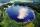Across the circle lakepasses through its center bridge over the lake. At three different locations on the lake shore are three fishermen A, B, C. Which of fishermen see the bridge under the largest angle?
3. SectorThe perimeter of a circular sector with an angle 1.8 rad is 64 cm. Determine the radius of the circle from which the sector comes.
4. PrismThe base of the prism is a rhombus with a side 30 cm and height 27 cm. The height of the prism is 180% longer than the side length of the rhombus. Calculate the volume of the prism.
5. Hexagon ACalculate area of regular hexagon inscribed in circle with radius r=9 cm.
6. Flowerbed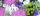On the flowerbed was planted 280 flowers - pansies and crayons. During the first week wilts quarter of pansies and an eighth crayons, which is 20% of all flowers. How many pansies were planted on the flowerbed?
7. Arc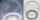The length of the circle is 41 amd arc length of the circle 9. What is the magnitude of the angle of this arc?
8. Blueberries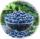Marie earns 3 liters of blueberries for 3.4 hours, Peter 2.4 liters for 2.9 hours. How long have earns together one liter?
9. RT 10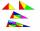Area of right triangle is 84 cm2 and one of its cathethus is a=10 cm. Calculate perimeter of the triangle ABC.
10. Cuboid diagonalCalculate the volume and surface area of the cuboid ABCDEFGH, which sides abc has dimensions in the ratio of 9:3:8 and if you know that the wall diagonal AC is 86 cm and angle between AC and the body diagonal AG is 25 degrees.
11. Scale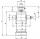Cylinder was drawn in scale 2:1. How many times is the volume of the cylinder smaller in reality?
12. Posters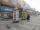Column with posters in the form of a cylinder is 2 m high and its diameter is 1.7 m. What is the content area for which it is possible to stick posters?
13. Rectangles - sidesOne side of the rectangle is 10 cm longer than second. Shortens longer side by 6 cm and extend shorter by 14 cm increases the area of the rectangle by 130 cm2. What are the dimensions of the original rectangle?
14. Two valves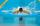Water fill the pool by two valves for 11 days. After 7 days the first valve was stopped and the second valve fill pool for 7 days. How many days took to fill the pool each of the valves individually?
15. Slope of the pool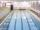Calculate slope (rise:run) of the bottom of swimming pool long 30 m. Water depth at beginning of pool is 1.13 m (for children) and depth at end is 1.84 m (for swimmers). Slope express as percentage and as angle in degrees.
16. Machines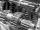In the workshop are three machines. The first is treated 250 parts for 4.7 hours, the second is treated 300 parts for 1.9 hours and the third is treated 230 parts for 4.6 hours. How long will take to make 3100 parts if worked all three machines at the
17. Three workers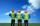Three workers A, B, C have to work on a specific task. Workers A and B completed whole task in 14 days, B together with C for 23 days, A together with C for 13 days. How long it would take to complete the task every one of them alone? How long it would
18. Two runners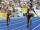Two runners ran simultaneously towards each other from locations distant 34.6 km. The average speed of the first runner was 1/5 higher than the average speed of the second runner. How long should each ran a 34.6 km, if you know that they meet after 67 m
19. Mushrooms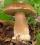Fresh mushrooms contain 94% water, dried 14%. How many kg of fresh mushrooms is needed to collect to get 10 kg dried?
20. OPT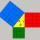What is the perimeter of a right triangle with the legs 14 cm and 21 cm long?

Do you have an interesting mathematical word problem that you can't solve it? Submit math problem, and we can try to solve it.

We will send a solution to your e-mail address. Solved examples are also published here. Please enter the e-mail correctly and check whether you don't have a full mailbox.

Please do not submit problems from current active competitions such as Mathematical Olympiad, correspondence seminars etc...